## Math and Scala with a Little Bit of Clojure

Martin Odersky is teaching Functional Programming Principles in Scala on Coursera and I attempted to complete this course, I completed the first assignment on recursion before the hard deadline which was hard given my work schedule at the time, however, I decided not to submit it, I remembered how much I hate school, or at least being a student in a school even a virtual one, I have my own timeline and my own interests.  While doing the problems I realized, and I know this is not a major revelation, that these first three problems are set in the context of math.  However, some of that math may not be immediately obvious to everyone so I thought it would make a nice post.  I was originally going to publish my solutions in this post, after the hard deadline of course, but there was recently some unpleasantness about publishing solutions and cheating and it seems that it is still possibly a violation of the honor code.  I feel having some code for two of the problems increases the quality of this posting so I will provide my examples for those solutions in Clojure, the non solution code will be in Scala so it’s now a hybrid posting.  Also the ideas for this post forced me to finally write my previous post on Pascal’s Triangle, which this post will draw on.

### Exercise 1: Pascal’s Triangle

The first problem is to recursively compute a binomial coefficient within Pascal’s triangle, this is fairly trivial if you have exposure to recurrence relations and recognize it as such, it is expressed as follows:

Some other common recurrence relations that are popular in CS educational circles include, Fibonacci numbers, triangular numbers and of course the factorial function these are expressed as:

I chose a term based representation of the factorial function over the more traditional:

These can be implemented recursively in Scala as:

def fibonacci(n : Int) : Long = {

if(n <= 1)

1

else

fibonacci(n - 1) + fibonacci(n - 2)

}

def triangularNumber(n : Int) : Long = {

if(n <= 1)

1

else

n + triangularNumber(n - 1)

}

def factorial(n : Int) : Long = {

if(n <= 1)

1

else

n * factorial(n - 1)

}

All of the above examples are inefficient and in many cases considered to be naïve solutions.  The Fibonacci example is terrible as it incurs an exponential number of function calls, fortunately there ways to optimize these algorithms.  One potential approach would be to use the closed formulas for triangular numbers or Binet’s formula for Fibonacci numbers.  I will ignore those as I have covered them previously and they are not what I am going for here.  I want to look at two optimizations, one is a way is the use of the tail call optimization and the second is to reduce the number of function calls.

Tail call optimization, put simply, is a mechanism that allows certain recursive functions that are written in a tail recursive form to be converted to iteration when they are executed.  It is my understanding that this requires some extra work in the JVM, at least in the older versions, I think 7 and 8 makes changes for this.  So this extra work requires some special syntax beyond simply putting your code in tail recursive form, Lisp and Scheme for example do this automatically, in Scala this requires the @tailrec annotation, using this form our functions get converted as follows:

def triangularNumber(n:Int) : Long = {

@tailrec

def triangularNumberAcc(acc: Long, n: Int): Long = {

if (n <= 1) acc

else triangularNumberAcc(n + acc, n - 1)

}

triangularNumberAcc(1, n)

}

def factorial(n:Int) : Long = {

@tailrec

def factorialAcc(acc: Long, n: Int): Long = {

if (n <= 1) acc

else factorialAcc(n * acc, n - 1)

}

factorialAcc(1, n)

}

As you can see the tail recursive forms take on an accumulator parameter.  The other optimization  I mentioned was to get rid of the extra function call for the Fibonacci, this is done by calculating the series using a second accumulator parameter, so that term Fn and term Fn+1 are passed as accumulator parameters.   This following example came out really nice as the base function sequenceGenerator can also generate the Lucas numbers if seeded appropriately:

@tailrec

def sequenceGenerator(n: Int, t0: Long, t1: Long): Long = {

if(n <= 0)

t0

else

sequenceGenerator(n - 1, t1, t0 + t1)

}

def fibonacci(n : Int) : Long = {

sequenceGenerator(n, 0, 1)

}

def lucas(n : Int) : Long = {

sequenceGenerator(n, 2, 1)

}

Going back to Pascal’s Identity if we look at the example from my last post:

We can represent the values that get calculated for this example visually as:

This gives some sense of how the recursive solution moves up through the triangle to sum the values, the example code, in Clojure, the naïve solution is:

(defn bc [r c]

(if (or (<= c 0) (<= r 0) (<= r c))

1

(+ (bc (dec r) (dec c)) (bc (dec r) c))

)

)

Additionally the Clojure version of our Binomial Coefficient can be similarly single call/tail call optimized:

(defn bc [rr cc]

(loop [r rr c cc acc 1]

(if (or (= c 0) (= r 0) (= r c))

acc

(recur (dec r) (dec c) (/ (* acc r) c)))

)

)

As mentioned most of these have closed form formulas like Binet’s formula for Fibonacci numbers.  Also if we take the formula for the binomial coefficient and since r ≥ c we can get the following cancelation of c!:

Which can be implemented in Scala, using the factorial function defined above, as:

def partialFactorial(s : Int, e: Int) : Long = {

@tailrec

def partialFactorialAcc(s : Int, e: Int, acc: Long): Long = {

if(e >= s) acc

else  partialFactorialAcc(s - 1, e, s * acc)

}

partialFactorialAcc(e, s, e)

}

def binomialCoefficient(r : Int , c : Int) : Long = {

if((c <= 0) || (r <= 0) || (r <= c))

1

else

partialFactorial(r, c+1)/factorial(r-c)

}

Both of these two “optimized” solutions for the binomial coefficient introduce division which can be problematic due to remainders and floating point rounding errors this would also be the case for the closed form approaches to calculating these numbers.  There is a variant for the binomial coefficient that is discussed in a paper which uses a gcd calculation to try to deal with these issues.

Another interesting observation in terms of how values are calculated between the naïve recursive approach and the tail recursive accumulator approach is that there seems to be some order duality on how the calculations are performed.  In the naïve approaches the recursion goes to the bottom and accumulates as it comes back up in the call stack.  In the accumulator versions the values are accumulated on the way down and returned from the bottom of the call stack.

### Exercise 2: Parentheses Balancing

The next problem is not so much about the solution as it is about the combinatorial properties of the input values.  The problem was to take a string and determine if the parenthesis in the string matched, this is also probably pretty common CS educational fodder.  So there will be no code for this problem.

The math that we are interested in here relates to the central binomial coefficient, it is a number sequence known as the Catalan numbers after the Belgian mathematician Eugène Charles Catalan.  It is given by the following equations:

In the original problem the text containing parentheses was the input, since a parenthesis counting algorithm would need to filter the text or implement some type of get next parenthesis function, we will assume that we have a string that only has only parenthesis.  First thing to notice that valid input strings need to be of even length.  Each term of the Catalan numbers describes how many possible sets of matching parenthesis you can have for each number n.  The table below illustrates the first five possibilities:

 n Cn Matching parentheses set Total String Size 22n n=0 1 way λ 0 n=1 1 way () 2 n=2 2 ways ()(), (()) 16 n=3 5 ways ()()(), ()(()), (())(), (()()), ((())) 64 n=4 14 ways ()()()(), ()()(()), ()(())(), ()(()()), ()((())),(())()(), (())(()), (()())(), ((()))(), (()()()),(()(())), ((())()), ((()())), (((()))) 256 n=5 42 ways ()()()()(), ()()()(()), ()()(())(), ()()(()()), ()()((())),()(())()(), ()(())(()), ()(()())(), ()((()))(), ()(()()()),()(()(())), ()((())()), ()((()())), ()(((()))), (())()()(),(())()(()), (())(())(), (())(()()), (())((())), (()())()(),(()())(()), ((()))()(), ((()))(()), (()()())(), (()(()))(),((())())(), ((()()))(), (((())))(), (()()()()), (()()(())),(()(())()), (()(()())), (()((()))), ((())()()), ((())(())),((()())()), (((()))()), ((()()())), ((()(()))), (((())())),(((()()))), ((((())))) 1024

The table above also includes the number of possible strings with only the two parentheses, which grows at a rate 22n.  This table is based on one from a paper by Tom Davis on Catalan numbers.  It is quite interesting paper as Catalan numbers also describe diagonal avoiding paths, “mountain ranges”, noncrossing partitions, multiplication orderings, the number of different ways a convex polygon with n + 2 sides can be cut into triangles, to mention a few.  Another interesting thing about Catalan numbers is they can be described by a recurrence relation:

I confess that this may not be that pragmatic in terms of solving the problem, but I think it is interesting and possibly gives a larger context to the problem.

### Exercise 3: Counting Change

The last problem, which is probably something of a CS classic problem as it appears in SICP, is the problem of counting change, given some numerical amount and some set of coin denominations we need to count the number of ways that we can represent the amount given the set of denominations. I confess to having cheated a little bit, well ok a lot, I found this post by Bill the Lizard. I also found this post with a more elegant solution and translated these into both Scala and Clojure, well ok I guess it’s not cheating since I didn’t submit it.  The clojure version is:

(defn count-change [amount coin-values]

(cond (= amount 0) 1

(or (< amount 0) (empty? coin-values)) 0

:else

(+ (count-change amount (rest coin-values))

(count-change (- amount (first coin-values)) coin-values))

)

)

The math here is from number theory and combinatorics, actually it might fall under combinatorial number theory.  In number theory there are two pretty big areas, one is multiplicative number theory which dates back at least to the Greeks and deals with how numbers divide and remainders and prime numbers which includes things like the well known fundamental theorem of arithmetic.  A relatively newer area of number theory is known as arithmetic number theory and is mostly attributed to Euler. In fact it was Euler who initiated the study of number partitions in his book Introduction to the Analysis of the Infinite in 1748.  It is the math of number partitions that our change problem falls under.   We are basically counting a subset of the partitions of a number’s full partition set.  In fact we can create a function that uses a Clojure list comprehension to create a number partition function from our change counting function:

(defn number-partition [n]

(count-change n (for [x (range 1 (inc n))] x ))

)

Functions to count partitions have been studied over the years and Euler invented a generating function that gives rise to, you guessed it, a recurrence equation to count partitions:

### School’s out

So there it is some (hopefully) interesting math context for these problems. I guess it’s kind of a gray area but I am pretty sure I didn’t cross the line on the whole honor code thing but even if I did I believe it’s covered by the People's Freedom of Choices and Voices Act.

Well it was a fun post to write, I got some code in a post, something I haven't done lately, which is only fitting for a blog called Elegant Coding, I think it came out pretty decent but remember I am still learning Scala and Clojure so my code examples here may not be as elegant as they could be.  I guess another objective of this post and my blog in general is to explore ways to mix programming and math education, while I think this post makes for a nice start along these lines, it could probably be improved which would be nice.

## 08 October 2012

### The Ubiquitous Patterns of Pascal’s Triangle

Pascal’s Triangle is one of the most fascinating and intriguing objects in math, I was first introduced to it in the sterile context of high school algebra as an aside.  Only decades later did I discover its true grandeur when I rediscovered it in the context of combinatorics and number theory.  I will attempt to provide some, hopefully, enlightening images but I feel that mathematical notation is both important and beautifully concise and allows one to have a transcendent experience with math so we will start with a formula, one of my “Eleven Equations”. The more I learn the more I feel it is more than justified to be in that list. I also feel that its centrality in combinatorics and probability theory makes it an equation that every math or computer science geek or enthusiast should be able to both recite off of the top their heads and be able to apply, if you don’t agree maybe this post will inspire you to think differently.  The equation is that of the binomial coefficient formula and it describes each element of Pascal’s Triangle:

The above formula evaluates under the rules that c ≤ r,  r ≤ 0 and 0! = 1.  I prefer the use the letters r and c over the traditional n and k as in n chose k, one can as easily say r choose c.  The reason I prefer r and c is that r means row and c means column and these values can be viewed as a way to address positions within Pascal’s Triangle, for example using the binomial coefficient notation from the above formula Pascal’s Triangle can be represented as:

As you can see in the above representation each position is the address (r,c) given by the coefficient notation.  Evaluating the expressions above with the Binomial Coefficient formula yields the more common representation of Pascal’s Triangle:

### Binomial Theorem

If I recall correctly and has been a while, the high school algebra introduction to Pascal’s triangle was mostly tied to the Binomial Theorem, which is written with sigma (summation) notation as:

For our purposes we are interested in the one variable version which can be written as:

This can be expanded to:

This can be written in "Pascal’s Triangle Form" as:

These types of introductions often focus on the additive properties as well. Each element is the sum of the two elements above it, which can be illustrated as:

The logic of this representation assumes that there is a zero on each side to add to propagate the ones on both side, we can rewrite this using the binomial coefficient notation as:

I admit this is where it starts to get more interesting to me. Not to denigrate its importance but I never really got that excited by the whole binomial theorem thing, although the series e stuff is pretty cool.  If we pick 5 choose 3 in the above representation, it can be written as the following sum:

If you look at the above representation you can see that this can be done for all entries that have two parents, this can be generalized as:

This formula, which is a recurrence relation, is known as Pascal’s Identity, it is one of several formulas known as Combinatorial Identities.

### Powers of Two

Another interesting and extremely important pattern in Pascal’s Triangle is the fact that the values of each row sum to the power of two of the row number, which the following representation depicts:

This can be written as the following formula, I think this also qualifies as an identity:

### Sets

As I mentioned at the beginning the binomial coefficient formula is read as r choose c.  I was trying to get back to that but I took the long way, so looking at our examples above our 5 choose 3 means if I have five things how many ways can I choose three of them which is 10 ways .  This is useful for counting, in combinatorics and probability theory I could take a deck of 52 cards and count how many 5 card hands I could choose and calculate probabilities of getting various hands, these are pretty standard applications of these types of counting problems.  However, if we step back and look at this counting, where each row represents a set of items, then each column represents the number of subsets of size c, this another reason I prefer c here it means both column and cardinality of the subset.  We can create a representation of Pascal’s Triangle to show this, but it does become unwieldy pretty quickly due to the exponential growth of each row, we would get:

In this representation each number represents a set element, these can map to any sets with these cardinalities.  Each column is wrapped by parentheses.  The ones on the left are created by the empty set and the ones on the right are created by the set itself.  So each row in Pascal’s triangle gives the structure of the powerset of a set with the cardinality of the row number.  Also it is not a coincidence that each row sums to a power of two since the cardinality of the powerset is the cardinality of the set raised to the power of 2.

### Powerset Lattice

Since I’ve had lattice theory on my mind lately, I couldn’t help but calling on our old friend the powerset lattice, as you can see in the context of the above each powerset lattice is going to have a row from Pascal’s triangle imprinted on it, each antichain consists of sets of the same cardinality and the number sets in the antichain maps back to a column:

### Principle of Inclusion and Exclusion (P.I.E)

The principle of inclusion and exclusion is used to count the size (cardinality) of a union of sets based on the cardinality of the sets and their intersections, the most common form, which appears in many probability texts, is:

|A ∪ B| = |A| + |B| - |A ∩ B|

The reason for this is that if you take the size of the union two sets, if they have any common elements then they counted twice, so subtracting the intersection removes the duplicate count.  This type of problem, counting things more than once and then needing to remove the duplicates comes up in other counting problems as well.  The venn diagram below shows the intersection of two sets, these are the elements that get counted twice in |A| + |B|:

Now as a disclaimer this Pascal’s triangle correlation is strictly my own observation so it might be wrong, but it seems to hold.  If you take the formula from above and add a term for the empty set, which is not needed as it evaluates to zero you get |∅| + |A| + |B| - |A ∩ B|.  Now you can see the number of elements for each term with number of sets (0, 1, 2) are 1 2 1, row 2 of Pascal’s triangle, for three you have 1 3 3 1:

 |A ∪ B ∪ C| = |∅| + (1 |A| + |B| + |C| (3 - |A ∩ B| - |A ∩ C| - |B ∩ C| (3 + |A ∩ B ∩ C| (1

And for four we have (1 4 6 4 1)

 |A ∪ B ∪ C ∪ D | = |∅| + (1 |A| + |B| + |C|+ |D| (4 - |A ∩ B| - |A ∩ C| - |A ∩ D| - |B ∩ C| - |B ∩ D| - |C ∩ D| (6 + |A ∩ B ∩ C| + |A ∩ B ∩ D| + |A ∩ C ∩ D| + |B ∩ C ∩ D| (4 - |A ∩ B ∩ C ∩ D | (1

This does not prove it but I would bet the pattern continues.  Like I said this is my own observation, so it might be wrong, if you know either way please let me know.

### Binomial Distribution

The binomial distribution is given by the following formula:

I converted it to my r,c nomenclature, I may be going too far using it here but I wanted to keep consistent, remember r=n, and c=k.  It is a discrete probability distribution for the number of successes of r independent yes/no experiments each of which yields success with probability p. If you set p=1/2, the symmetric case, then the row number r divided by 2r gives you the binomial distribution. By the central limit theorem this symmetric case approaches the normal distribution as r increases.

### Central Binomial Coefficient and Symmetry

The above representation shows the symmetry in Pascal’s Triangle, each row is colored in a way that highlights the numbers that are the same, the first and last columns consisting of ones match each other obviously, but in each row the second column matches the second to last column, and the same for the third column and so one. This gives rise to, you guessed it, another combinatorial identity:

The columns highlighted in the gray box are special case called the central binomial coefficient. If you notice each even numbered row has an odd number of columns with the central one only occurring only once, it still follows the above identity but the identity evaluates to a value equaling itself.  The central binomial coefficient is given by the following form, I use n here since we don’t care about row/column in this case:

### Figurate Numbers

There is an area of math that deals with figurate numbers, these are numbers formed by arranging dots to form various polygons which form sequences as you expand size of each polygon, I looked at some interesting properties of triangular numbers in a previous post, for example here are the first 5 triangular numbers:

Triangular numbers are created by adding up the natural numbers, but there is a binomial formula which evaluates to a closed formula:

The idea of figurate numbers can be extended into higher dimensions, so not only do you have numbers that create polygons you can create polyhedrons as well.  When you add up triangular numbers, you get the tetrahedral number which can be described by an analogous formula:

This can be visually depicted as stacking up the triangular number to form tetrahedrons:

Pascal’s Triangle has a number of relations with figurate numbers, but the most notable are the triangular and tetrahedral numbers, shown in red and blue respectively:

Also if you look at the binomial expression for these you will notice that the column is fixed, for triangular numbers it is c=2 and for tetrahedral numbers it is c=3, this maps our addressable form to the second and third columns as shown.

The above tabular form is based on a table from Concrete Mathematics, 2nd Edition by Ronald L. Graham, Donald E. Knuth and Oren Patashnik.  Again you can see column one, where c=1, is the natural numbers, column two is the trianglular numbers, the summation of natural numbers and three is the tetrahedral numbers, the summation of the triangular numbers.  This would imply that this pattern continues to progress, and it does. The next column are the pentatope numbers, which describe a series of four dimensional polytopes, a polytope is an extension of a polyhedron into higher dimensions, called pentachorons which are formed by stacking three dimensional tetrahedrons in four dimensions. These numbers are given by the formula:

And these numbers progress into higher dimensions, each summing the numbers immediately below them. These are generalized as the pyramidal numbers and are described by the following table:

 Number Summation Form Binomial Form Closed Form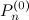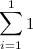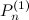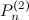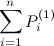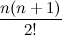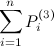### Powers of Eleven

There is another interesting pattern in Pascal’s Triangle each successive row is equivalent to a power eleven of as you can see in the following table:

 0 110 1 1 111 11 2 112 121 3 113 1331 4 114 14641 5 115 161051

Oops, I fibbed a little here, 115 does not equal the row (1 5 10 10 5 1), this breaks down due to the need to carry 1 to make ten. However, the pattern will hold if you push up the number base, for example 11 hexadecimal, which I will write as 1116, to the 5th power is (1116)5 = 15AA51, remember A equals 10 so now the pattern holds, and it will continue to hold as long as you keep upping the number base, but remember (1116)5≠ 115.

### Sierpinski Triangle

There is another interesting relation to another mathematically interesting triangle, the Sierpinski Triangle aka Sierpinski gasket is a fractal that can be constructed by taking an equilateral triangle and removing the center which is an equilateral triangle of ¼ area and then repeating this on each of the three remaining triangles, and so on.  It can also be created by applying modulo 2 to each element in Pascal’s Triangle as shown below. The zeros are grayed out to highlight the pattern:

### And the List Goes On...

As we saw we ventured into several areas of mathematics, combinatorics, number theory, probability theory, fractal geometry and geometry (topology), and lattice theory.  There are many other patterns such as relations to polytopes, cellular automata, a pattern with prime numbers and more.  The Wikipedia page has many interesting patterns, some of which I used here also two other interesting sites are Christopher Olah’s post and this.